# CamelCase

Camel case is a style of writing compound words or phrases with elements or words written together, without spaces or other punctuation. Each word’s first letter is capitalized, and the rest of the word is written in lower case.

The task is: read a user-entered string (possibly with spaces, digits and punctuation), remove from it all non-letter characters and write the result in camel case.

This type of examples shows string manipulations: reading, modifying etc.

Test examples:

“Test Example One” -> “TestExampleOne”
” exampleTwo ” -> “Exampletwo” (case of original words doesn’t matter)
“!!! is_this_3RD EXAMPLE?..” -> “IsThisRdExample” (digits and punctuation are considered to be delimiters)

### Example for versions perl 5.12.1, perl 5.8.8

``````my \$text = <STDIN>;
\$text = join('', map(ucfirst, split(/[^a-z]+/, lc \$text)));
print \$text, "\n";
``````

### Example for versions clisp 2.47, Corman Common Lisp 3.0, SBCL 1.0.1, SBCL 1.0.29

``````(defun camel-case (s)
(remove #\Space
(string-capitalize
(substitute #\Space nil s :key #'alpha-char-p))))

``````

### Example for versions Python 2.6.5

The program uses Python standard library functions `translate` and `title`.

`title` counts all non-letters as word delimiters, so no need to change them to spaces before calling `title`.

``````non_letters = ''.join(c for c in map(chr, range(256)) if not c.isalpha())

def camel_case(s):
return s.title().translate(None, non_letters)

print camel_case(raw_input())
``````

### Example for versions Groovy 1.7, Sun Java 6

This example uses Java regular expressions. A regular expression `[a-zA-Z]+` describes any contiguous sequence of letters (in any case), surrounded with non-letter characters or ends of string. Classes `Pattern` and `Matcher` allow to create this regular expression and extract from the string all fragments which match it. For each such fragment, its first letter is converted to upper case, and the rest of it — to lower case. Finally, the resulting word is appended to `StringBuffer` variable which accumulates the result.

``````import java.util.regex.*;
import java.io.*;

public class CamelCase {
public static void main(String[] args) {
try {
Pattern p = Pattern.compile("[a-zA-Z]+");
StringBuffer result = new StringBuffer();
String word;
while (m.find()) {
word = m.group();
result.append(word.substring(0, 1).toUpperCase() + word.substring(1).toLowerCase());
}
System.out.println(result.toString());
} catch (Exception e) {
System.err.println("An error occured while reading input string.");
}
}
}
``````

### Example for versions vbnc 2.4.2

This program checks each letter of the string for being a letter; if it is not, it is replaced with a space. After this the string is converted to proper case (all words are lower case, starting with capital letter), and finally all spaces are removed.

``````Module Module1
Sub Main()
Dim Text As String
Dim i As Long

Try
Catch ex As Exception
Console.WriteLine("Invalid input.")
Return
End Try

For i = 1 To Len(Text) Step 1
If InStr("abcdefghijklmnopqrstuvwxyz", GetChar(Text, i)) = 0 Then
Text = Replace(Text, GetChar(Text, i), " ")
End If
Next
Console.WriteLine(Replace(StrConv(Text, vbProperCase), " ", ""))
End Sub
End Module
``````

### Example for versions SpiderMonkey 1.7

This example is meant to be executed from web-browser, same as quadratic equation. Input form should look like this:

``````<form name="CamelCase">
<input type="text" required="required" name="txt">
<input type="button" value="Convert to CamelCase" onClick="convert()">
</form>
``````

The code itself could have been written in one line, but has been broken in several parts for better readability. First line gets the string to process; second line converts it to lower case and replaces all non-letter characters with spaces; third line capitalizes each word; and fourth line removes all spaces. JavaScript has a very strong support of regular expressions, so this is done easily.

``````function convert() {
txt = document.CamelCase.txt.value;
txt = txt.toLowerCase().replace(/[^a-z ]+/g, ' ');
txt = txt.replace(/^(.)|\s(.)/g, function(\$1) { return \$1.toUpperCase(); });
txt = txt.replace(/[^a-zA-Z]+/g, '');
document.getElementById('output').innerHTML = txt;
}
``````

### Example for versions Borland C++ Builder 6, g++ 3.4.5, Microsoft Visual C++ 9 (2008)

This example is based on character-by-character string processing.

`getline` reads a string (delimited with end of line) from argument stream. Function `tolower` works only with single characters, so to convert whole string to lower case it is used with `transform` function. The latter applies `tolower` to all elements in the range `[text.begin(), text.end())` and stores the results in a range starting with `text.begin()` again.

After this the string is processed char-by-char. Each character is checked for being alphabetic; if it is, it is appended to the resulting string (converted to upper case if previous character was non-alphabetic); if it is not, it only affects `lastSpace` (which is true only if last character was non-alphabetic).

`isalpha` works with both uppercase and lowercase letters, so it was possible not to convert the input string to lowercase, but rather to convert each appended character.

``````#include <string>
#include <iostream>
#include <algorithm>

using namespace std;

int main() {
string text, cc="";
bool lastSpace = true;
getline(cin, text);
transform(text.begin(), text.end(), text.begin(), (int (*)(int))tolower);
for (int i=0; i<text.size(); i++)
if (isalpha(text[i])) {
if (lastSpace)
cc += toupper(text[i]);
else
cc += text[i];
lastSpace = false;
}
else {
lastSpace = true;
}
cout << cc << endl;
return 0;
}
``````

### Example for versions gcc 3.4.5, gcc 4.2.4, tcc 0.9.25

This example is based on character-wise string processing. `fgets` here reads at most 99 characters into the string, and stops when it finds end-of-string character, so a long line might be split. C doesn’t provide boolean data type, so it has to be simulated using integer variable.

``````#include <stdio.h>

void main() {
char text,cc;
fgets(text, sizeof text, stdin);
int i,j=0,lastSpace=1;
for (i=0; text[i]!='\0'; i++)
if (text[i]>='A' && text[i]<='Z' || text[i]>='a' && text[i]<='z')
{   if (lastSpace>0)
cc[j] = toupper(text[i]);
else
cc[j] = tolower(text[i]);
j++;
lastSpace = 0;
}
else
lastSpace = 1;
cc[j]='\0';
printf("%s\n",cc);
}
``````

### Example for versions gcc 3.4.5 (Objective-C)

``````#import <Foundation/Foundation.h>

NSString *camelCase(NSString *s) {
return [[[s capitalizedString] componentsSeparatedByCharactersInSet:[[NSCharacterSet characterSetWithCharactersInString:@"ABCDEFGHIJKLMNOPQRSTUVWXYZabcdefghijklmnopqrstuvwxyz"] invertedSet]] componentsJoinedByString:@""];
}

int main (int argc, const char * argv[]) {
NSAutoreleasePool * pool = [[NSAutoreleasePool alloc] init];

NSLog(@"%@", camelCase(@"Test Example One"));
NSLog(@"%@", camelCase(@"exampleTwo "));
NSLog(@"%@", camelCase(@"!!! is_this_3RD EXAMPLE?.."));

[pool drain];
return 0;
}
``````

### Example for versions Oracle 10g SQL, Oracle 11g SQL

This example uses Oracle SQL regular expressions. First use of `regexp_replace` replaces all digits with spaces — this is necessary for further `initcap` usage (this function treats digits as parts of the words and doesn’t capitalize following letters). Next, `initcap` converts all words to lowercase with first letter capitalized. Finally, second use of `regexp_replace` removes all punctuation and spaces from the string.

&TEXT is substitution variable, which allows to input different strings without rewriting the select statement.

``````select regexp_replace(initcap(regexp_replace('&TEXT', '[[:digit:]]', ' ')), '([[:punct:] | [:blank:]])', '')
from dual
``````

### Example for versions Free Pascal 2.2.0, gpc 20070904, Turbo Pascal 4.0, Turbo Pascal 5.0, Turbo Pascal 5.5, Turbo Pascal 6.0

This example processes the string char by char, and works with ASCII-codes to figure out whether they are lower- or uppercase letters. `ord` returns ASCII-code of a character, while `chr` converts given ASCII-code into a character. String capacity is omitted and thus set to 255 by default.

Note that in Turbo Pascal series this program works only with Turbo Pascal 4.0 and higher due to the fact that earlier versions didn’t have `char` datatype.

``````program Camelcase;

var
text, cc: string;
c: char;
i: integer;
lastSpace: boolean;

begin
lastSpace := true;
cc := '';
for i := 1 to Length(text) do
begin
c := text[i];
if ((c >= #65) and (c <= #90)) or ((c >= #97) and (c <= #122)) then
begin
if (lastSpace) then
begin
if ((c >= #97) and (c <= #122)) then
c := chr(ord(c) - 32);
end
else
if ((c >= #65) and (c <= #90)) then
c := chr(ord(c) + 32);
cc := cc + c;
lastSpace := false;
end
else
lastSpace := true;
end;
writeln(cc);
end.
``````

### Example for versions Free Pascal 2.2.0, gpc 20070904, Turbo Pascal 4.0, Turbo Pascal 5.0, Turbo Pascal 5.5, Turbo Pascal 6.0

This example is similar to previous one, but uses sets of characters for letter check. This makes the code more readable.

Note that in Turbo Pascal series this program works only with Turbo Pascal 4.0 and higher due to the fact that earlier versions didn’t have `char` datatype.

``````program Camelcase;

var
text, cc: string;
c: char;
i: integer;
lastSpace: boolean;
upper, lower: set of char;

begin
upper := ['A'..'Z'];
lower := ['a'..'z'];
lastSpace := true;
cc := '';
for i := 1 to Length(text) do
begin
c := text[i];
if (c in lower) or (c in upper) then
begin
if (lastSpace) then { convert to uppercase }
begin
if (c in lower) then
c := chr(ord(c) - 32);
end
else { convert to lowercase }
if (c in upper) then
c := chr(ord(c) + 32);
cc := cc + c;
lastSpace := false;
end
else
lastSpace := true;
end;
writeln(cc);
end.
``````

### Example for versions gmcs 2.0.1

First line of `Main` method reads the input string from console and converts it to lowercase. Second line replaces all sequences of 1 or more non-alpha characters with spaces. Two next lines get object of class TextInfo and use it to convert the string to title case (all words start with capital letters). Finally, spaces are removed from the resulting string, and the result is written to console.

``````using System;
using System.Globalization;
using System.Text.RegularExpressions;

public class Program
{   public static void Main(string[] args)
text = Regex.Replace(text,"([^a-z]+)"," ");
TextInfo ti = new CultureInfo("en-US",false).TextInfo;
text = ti.ToTitleCase(text);
text = text.Replace(" ","");
Console.WriteLine(text);
}
}
``````

### Example for versions gmcs 2.0.1

This example uses only regular expressions. First pass replaces all maximum sequences of letters with result of applying CapitalizePart to them (i.e., makes first character uppercase). Second pass replaces all non-letters with empty string.

``````using System;
using System.Text.RegularExpressions;

class Program
{   static string CapitalizePart(Match m)
{   string x = m.ToString();
return char.ToUpper(x) + x.Substring(1, x.Length-1);
}
static void Main()
string cc = Regex.Replace(text, "([a-z]+)", new MatchEvaluator(Program.CapitalizePart));
cc = Regex.Replace(cc, "[^a-zA-Z]+", string.Empty);
Console.WriteLine(cc);
}
}
``````

### Example for versions Ruby 1.9

1. Read the string from standard input (`gets.chomp`)
2. Split the string into parts, separated with matches of regular expression, which describes a sequence of one or more non-letter characters
3. Apply to each part function `capitalise`
4. Concatenate the resulting strings (`join`) and print the result (`puts`)
``````puts gets.chomp.split( /[^a-zA-Z]+/ ).map {|w| w.capitalize}.join
``````

### Example for versions Ruby 1.9

This works in a same way as this example, but `scan` extracts the matches of regular expression instead of discarding them and extracting parts of string between them, as `split` does.

``````puts gets.chomp.scan( /[a-zA-Z]+/ ).map {|w| w.capitalize}.join
``````

### Example for versions Scala 2.8.0-final

This example uses regular expressions twice. First one, `words`, describes the words in the text; each match of it is replaced with itself, converted to lower case and capitalized. Second one, `separators`, describes the spaces between words; all matches of it are replaced with empty string, i.e., just removed from the string.

``````import java.io.{BufferedReader, InputStreamReader}
import scala.util.matching.Regex

object Main {
def main(args: Array[String]) {
val words = """([a-zA-Z]+)""".r
text = words.replaceAllIn(text, m => m.matched.toLowerCase.capitalize)
val separators = """([^a-zA-Z]+)""".r
text = separators.replaceAllIn(text, "");
println(text);
}
}
``````

### Example for versions Scratch 1.4

Since Scratch is a graphical language, the screenshot contains all the actual information about the program.

This example can’t be done using only standard blocks provided by the language; to implement it, we’ll need to explore hidden possibilities of the environment. Two blocks which handle conversions between ASCII codes and letters can be added as described in this tutorial on Scratch web-site. Once they are available, the rest of the program is quite evident.

Note that arrays are indexed starting with 1, and boolean values have to be compared with `true` and `false` explicitly to produce a condition.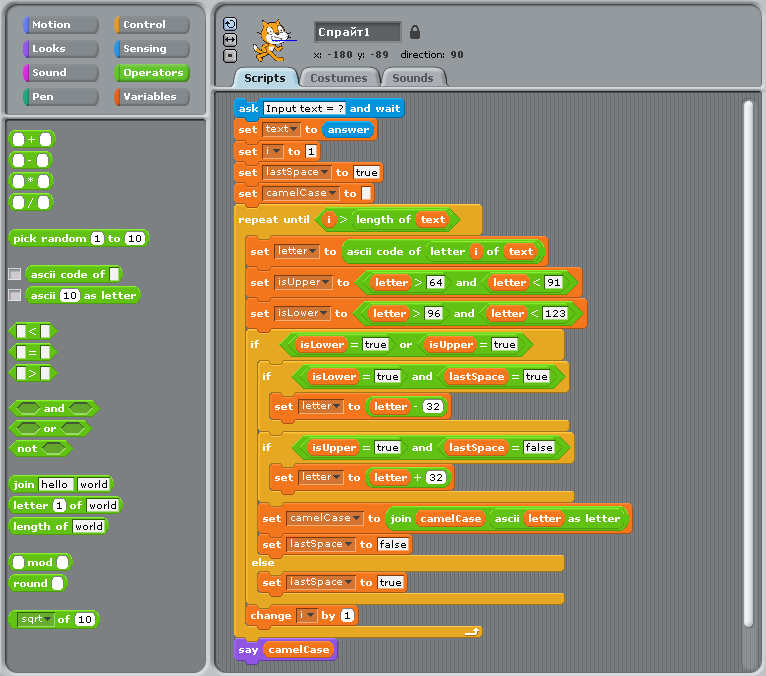CamelCase example in Scratch

### Example for versions j602

`alpha` is the set of upper and lower case alphabetic characters.

`camel` is an explicit solution to the problem, meaning that the variables to the function(`y`) are mentioned in the function body. The strategy used is to calculate the indices(`i`) of the alphabetic characters from the bit vector(`b`) which represents whether or not a character is alphabetic. The function then takes the leading index of each interval of these alphabetic characters and uses that to replace them by their capitalized form. Finally the string is sieved using `b` so that it contains only the alphabetic characters.

`camel2` is a tacit definition, it cuts the input string at the delimiters(non-alphabetic characters), leaving a collection of boxed strings. The leading character of each of these boxed strings is then capitalized and they are all concatenated together forming the final result.

`camel3` takes advantage of the built-in libraries, a regular expression is used to split the string into boxed strings, each of these is then capitalized and concatenated.

In all three functions the input is first converted to lower case.

`check` can be used to ensure that all the functions produce equivalent output.

``````alpha=: a.{~,65 97+/i.26

camel=: 3 : 0
y=. tolower y
i=. I. (> 0,}:) b=. y e. alpha
(b # toupper@(i&{) i} ]) y
)

isalpha=: e.&alpha
capital=: (toupper@{.,}.)^:(1<:#)
format =: [: ,&' ' tolower
case   =:  [: ; [: capital&.> ] <;._2~ [: -. isalpha
camel2 =: case@format

require'regex text'
camel3=: [: ; [: capitalize&.> [: '[a-zA-Z]+'&rxall tolower

check=: [: ,. camel;camel2;camel3
``````

### Example for versions Sanscript 2.2

First flowgram converts user-entered text in lowercase and passes it into a character-wise processing loop. Second diagram uses `Split Text` block for extracting next character of the string, which is then compared with characters with ASCII-codes before a and after z, and the result of comparison is passed to selection block. Third diagram shows processing of letters: depending on the value of `lastSpace` either the letter or its uppercase equivalent are appended to the result, and `lastSpace` is set to `FALSE`. Fourth diagram shows processing of non-letter characters: it only sets the value of `lastSpace` to `TRUE`.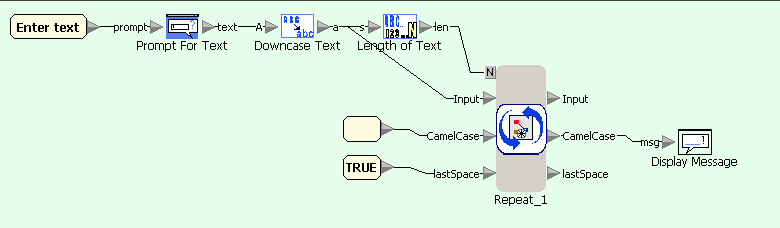CamelCase example in Sanscript (main flowgram)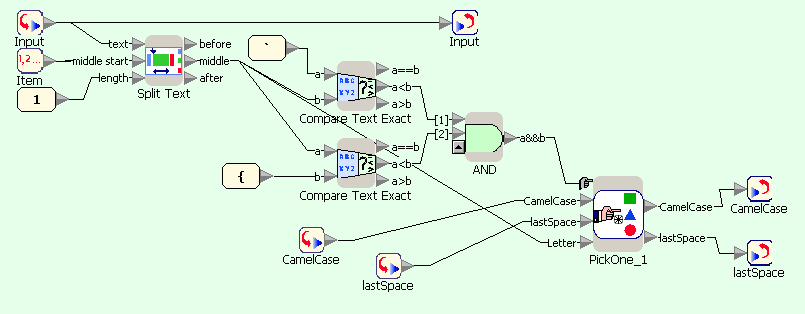CamelCase example in Sanscript (repeat block)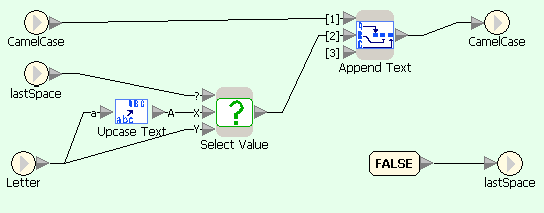CamelCase example in Sanscript (if character is letter)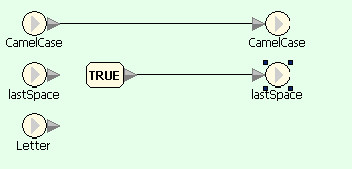CamelCase example in Sanscript (if character is not a letter)

### Example for versions PHP 5.3.2

This example uses string functions and regular expressions. Function `ucwords` converts first letter of each word to uppercase.

``````<?
\$text = fgets(STDIN);
\$text = str_replace(' ', '', ucwords(preg_replace('/[^a-z]/', ' ', strtolower(\$text))));
echo \$text;
?>
``````

### Example for versions Baltie 3

This example features a standard character-wise string processing. Note that the language doesn’t support boolean variables, so “previous character was a separator” is stored as an integer. Baltie 3 is a strongly typed language, so cast from character to its ASCII-code has to be done explicitly using a corresponding function.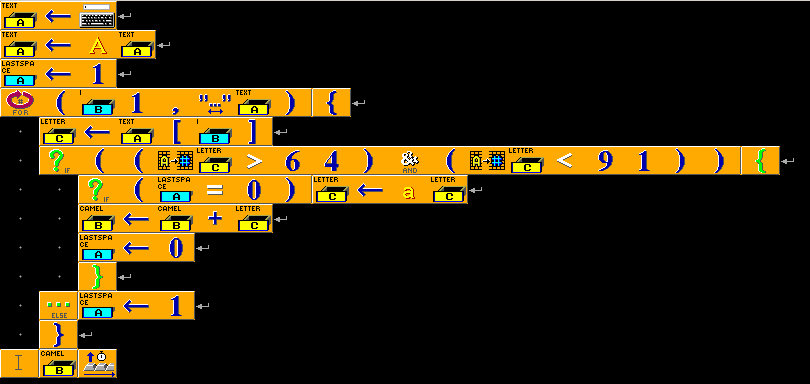CamelCase in Baltie 3

### Example for versions ActiveTcl 8.5, JTcl 2.1.0, Tcl 8.4, Tcl 8.5.7

This example shows character-wise string processing. A regular expression is used to check whether current character is a letter.

``````set S [gets stdin]
set S [string tolower \$S]
set L [string length \$S]
set lastSpace 1
set cc ""
for {set i 0} {\$i < \$L} {incr i} {
set letter [string index \$S \$i]
if { [string match {[a-z]} \$letter] } {
if { \$lastSpace == 1 } { set letter [string toupper \$letter] }
append cc \$letter
set lastSpace 0
} else {
set lastSpace 1
}
}
puts \$cc
``````

### Example for versions Io-2008-01-07

This example shows char-by-char string processing. Note that `at(i)` returns ASCII-code of i-th character of the string, and `slice(i,i+1)` returns the character itself as a string of length 1. The check whether the character is a letter is done using ASCII-codes, and conversion to uppercase and string output — using string representation.

``````S := File standardInput readLine asLowercase;
lastSpace := 1;
for(i,0,(S size)-1,
ascii := S at(i);
letter := S slice(i,i+1);
if(ascii>=97 and ascii<=122,
if(lastSpace==1, letter := letter asUppercase);
letter print;
lastSpace := 0,
lastSpace := 1;
);
);
``````

### Example for versions D2

First line reads a line from standard input and converts it to lowercase. Second line replaces all non-letter characters with spaces (last parameter is attribute characters; `g` means that all matches of regular expression are replaced, not only the first one). Third line capitalizes words in string, removes leading and trailing spaces and replaces all sequences of spaces with a single space. Finally, the spaces are removed from the string, and the result is printed to standard output.

``````import std.stdio;
import std.string;
import std.regexp;

void main() {
text = sub(text,"[^a-z]"," ","g");
text = capwords(text);
text = sub(text," ","","g");
writeln(text);
}
``````

### Example for versions Roco 20071014

The example is commented in detail. Coroutine `char` reads characters from standard input one by one and checks whether they are letters. Coroutine `letter` is called for characters which turned out to be letters; it converts them into required case and prints them. Note that `not` command inverts all bits of the number, so it can’t be used to negate a logical value (which is stored as 0 or 1) — one has to subtract this value from 1.

``````/*  - current character
 - last character was space?
rest are temporary variables (used within one iteration only)
*/

co letter{
/* coroutine to process the case of a known letter */
/* if it is uppercase, and last one was letter, change to lowercase */
sub  1 
and   
if 
/* if it is lowercase, and last one was space, change to uppercase */
and   
if 
sub   32
/* print the character */
cout 
set  0
ac
}

co char{
/* read next character to  */
cin 

/* break the loop when the next character is end-of-line (ASCII 10) */
eq   10
if  ac

/* check whether this character is a letter at all  - uppercase,  - lowercase,  - at all, - - temporary */
/* uppercase */
gt   64
lt   91
and   

/* lowercase */
gt   96
lt   123
and   

/* at all */
or   
sub  1 

/* if this is not a letter, ONLY change  */
if 
set  1
/* otherwise, call the coroutine to handle this */
if 
ca letter
}

/* at the start mark that last character was space */
set  1
ca char

ac
``````

### Example for versions gawk 3.1.6

Variable `\$0` stores the whole string read (as opposed to variables `\$1`, `\$2` etc. which store fields of the record). `split` splits the string into fragments which are separated with matches to the regular expression and writes the result to the array `words`. After this each element of the array is converted to correct case using functions `substr`, `toupper` and `tolower`.

``````{   text = \$0;
split(text, words, /[^a-zA-Z]+/);
for (i=1; i<=length(words); i++) {
res = res toupper(substr(words[i],1,1)) tolower(substr(words[i],2));
}
print res
}
``````

### Example for versions gawk 3.1.6, Jawk 1.02, mawk 1.3.3

mawk provides no function `length` to get the size of the array, neither it can be used in Jawk — an attempt results in “Cannot evaluate an unindexed array.” runtime error.

Instead we can use the fact that function `split` returns the number of string fragments it extracted from the string. Otherwise this example is identical to this one.

``````{   text = \$0;
N = split(text, words, /[^a-zA-Z]+/);
for (i=1; i<=N; i++) {
res = res toupper(substr(words[i],1,1)) tolower(substr(words[i],2));
}
print res
}
``````

### Example for versions Pike 7.8

This example implements character-by-character string processing. The only thing to note is that Pike provides no data type for characters. `text[i]` would return an integer — ASCII-code of the corresponding character. To get i-th character as a string variable, one has to use `text[i..i]` which is operation of extracting substring from a string.

``````void main()
{
string text = lower_case(Stdio.stdin->gets());
string cc = "";
int i, lastSpace = 1;

for (i=0; i<sizeof(text); i++)
{
if (text[i] >= 'a' && text[i] <= 'z')
{
if (lastSpace == 1)
cc += upper_case(text[i..i]);
else
cc += text[i..i];
lastSpace = 0;
}
else
lastSpace = 1;
}
write(cc+"\n");
}
``````

### Example for versions befungee 0.2.0

This program processes the input string character-by-character. The only instructions executed before the loop are `152p` — this puts value 1 in cell (5, 2) which will store 1 if the last character was not a letter, and 0 otherwise.

The main part of the program is the loop. One loop iteration reads (`~`) and processes one character of the input string. The loop breaks as soon as the character is its ASCII is 10 (`: 25*- #v_ @`), otherwise the instruction pointer goes to the second line (at `v` command). If the character is a lowercase letter, it is converted to uppercase to unify further processing (this is done by the part of the program before the first square block and the block itself). After this we check whether the letter is an uppercase letter. If it is not (pointer is back to line 1), we mark (5, 2) cell as 1, the character is removed from the stack, and the iteration is over. Otherwise we do one more check, this time on the value of (5, 2) cell, and depending on its outcome we keep the character or convert it to lowercase. Finally, the resulting character is printed, and the iteration is over.

``````152p > ~ : 25*- #v_ @               >48*-v                    >152p \$         v
> :: "`"` \"{"\` * |    > :: "@"` \"["\` * ! |    >    v
>    ^                    >52g |    >052p,v
>48*+^
^                                                                        <
( )
|
was last character a space (5,2)
``````

### Example for versions Whitespacers (Ruby)

``````push-1  { 	}
push-1  { 	}
save		 LOOP-START.label-0
{ }
push-2  { 	 }
push-2  { 	 }
push-10  { 	 	 }
subtract	  	if-0-goto-1
{	}
CONVERT-TO-LOWERCASE.duplicate
push-A  { 	     	}
subtract	  	if-neg-goto-2
{	 }
duplicate
push-Z  { 	 		 	 }
swap
subtract	  	if-neg-goto-2
{	 }
push-32  { 	     }
{	 }
CHECK-WHETHER-IS-LETTER.duplicate
push-a  { 		    	}
subtract	  	if-neg-goto-3
{		}
duplicate
push-z  { 				 	 }
swap
subtract	  	if-neg-goto-3
{		}
ACTION-IF-LETTER.CHECK-WHETHER-LAST-WAS-SPACE.push-1  { 	}
{	  }
push-32  { 	     }
subtract	  	label-4
{	  }
print
push-1  { 	}
push-0  {	 }
save		 goto-0

{ }
label-3
{		}
ACTION-IF-NOT-LETTER.push-1  { 	}
push-1  { 	}
save		 goto-0

{ }
label-1
{	}
push-10  { 	 	 }
print

end.memory:1-was-last-space,2-currentchar
``````

### Example for versions Morphett's FALSE

The input string is processed character by character. In Morphett’s FALSE strings are entered in a pop-up window, and end of input is marked by an empty line, which is then converted to `-1` value. That’s why `-1` is used for breaking the loop. Variable `s` stores “whether the previous character was a space” sign. Conditional commands execution (`?`) allows to run only `if-then` conditions; to implement `else` branch, one has to copy the condition (stored in variable `l` — whether the current character is a letter), invert it and use another `?`.

``````1_s: ^
[\$1_=~]
[ \$\$ 96> \123\> & [32-]?
\$\$ 64> \91\> & \$l: [s;~[32+]? , 0s:]? l;~[1_s: %]?
^]
#
``````

### Example for versions SML/NJ 110

``````val text = valOf (TextIO.inputLine TextIO.stdIn);
fun capitalize s = let
val (x::xs) = explode s
in
implode (Char.toUpper x :: map Char.toLower xs)
end;
val result = concat (map capitalize (String.tokens (not o Char.isAlpha) text));
print (result ^ "\n");
``````

### Example for versions perl 5.12.1, perl 5.8.8

This is similar to the previous example, except that instead of splitting on non-alphabetical characters, we match on runs of alphabetical characters.

``````my \$text = <STDIN>;
\$text = join('', map(ucfirst, lc(\$text) =~ /[a-z]+/g));
print "\$text\n";
``````

### Example for versions rakudo-2010.08

The first line reads the string to process, the second one — declares the variable which will store the result.

The most interesting things happen in the third line. The regular expression `<[a..zA..Z]>+` finds all words in the string, and for each found word the built-in code `{ \$cc ~= \$0.capitalize; }` is executed. It translates the word to the required case and appends it to the result.

``````my \$A = \$*IN.get;
my \$cc = "";
\$A ~~ s:g /(<[a..zA..Z]>+) { \$cc ~= \$0.capitalize; } //;
print \$cc;
``````

### Example for versions Seed7 2012-01-01

This example uses character-by-character processing.

``````\$ include "seed7_05.s7i";

const proc: main is func
local
var string: text is "";
var string: camel_case is "";
var char: ch is ' ';
var boolean: was_space is TRUE;
begin
text := lower(text);
for ch range text do
if ch in {'a' .. 'z'} then
if was_space then
ch := upper(ch);
end if;
camel_case &:= ch;
was_space := FALSE;
else
was_space := TRUE;
end if;
end for;
writeln(camel_case);
end func;
``````

### Example for versions Falcon 0.9.6.6

This program processes the input string character by character.

``````text = input().lower()
cc = ""
was_space = true
for i in [ 0 : text.len() ]
if text[i] >= 'a' and text[i] <= 'z'
if was_space
cc += text[i].upper()
else
cc += text[i]
end
was_space = false
else
was_space = true
end
end
printl(cc)
``````

### Example for versions iconc 9.4

First of all the program reads the string to process and adds a space to its end (`||` is concatenation operator). After this `text` variable is scanned. `?` is an operator which binds a string to the expression, so that all string matching functions in the expression are performed on this string.

Commands `ReFind` and `ReMatch` from regular expressions library `regexp` both find all sequences of symbols which match the given regex, but `ReFind` returns the index of sequence start, and `ReMatch` — the index of the first character after sequence end. In one iteration `ReFind` finds the start of the next sequence of non-letter characters. Command `tab` moves current position pointer to this position and returns part of the string from previous position of the pointer to the new position — a word. After this the word is converted to proper case and concatenated to the resulting string. `*word` is a function which returns length of the string. `map` replaces all characters of its first argument which are present in its second argument with corresponding characters from its third argument (in this case — switch case of characters; `&lcase` and `&ucase` are built-in constants which contain lowercase and uppercase alphabet). Finally, one more call of `tab` moves the pointer past the sequence of non-letter characters to the start of next word.

``````link regexp

procedure main ()
text := read() || " ";
cc := "";
text ? {
while j := ReFind("[^a-zA-Z]+") do {
word := tab(j);
cc ||:= map(word,&lcase,&ucase) || map(word[2:*word+1],&ucase,&lcase);
tab(ReMatch("[^a-zA-Z]+"));
}
}
write (cc);
end
``````

### Example for versions Factor 0.94

This example uses regular expressions. Word `re-split` (from `regexp`) splits a string into an array of strings separated by matches to the given regular expression. After this `map` combinator applies word `>title` (from `unicode.case`) to each element of the resulting array, converting them to title case. Finally, `join` (from `sequences`) concatenates the strings into one using “” as a separator.

``````USING: kernel io regexp sequences unicode.case ;

[ >title ] map
"" join print
``````

### Example for versions Oracle 11g SQL

This example uses Oracle regular expressions combined with PL/SQL. `regex_substr` returns a substring of `text` that is `occurrence`‘s match to the given regular expression.

``````declare
text varchar2(100) := '&user_input';
word varchar2(100);
camelcase varchar2(100);
occurrence number := 1;
begin
loop
word := regexp_substr(text, '[[:alpha:]]+', 1, occurrence);
exit when word is null;
camelcase := camelcase || initcap(word);
occurrence := occurrence + 1;
end loop;
dbms_output.put_line(camelcase);
end;
``````

### Example for versions loljs 1.1

Note that LOLCODE has no character processing functions, except for concatenation, so you can’t compare characters or get their ASCII-codes. This means that to figure out whether a character is a letter you need to create a “dictionary” of all letters and just look it up.

``````HAI
I HAS A UPP ITZ "QWERTYUIOPASDFGHJKLZXCVBNM"
I HAS A LOW ITZ "qwertyuiopasdfghjklzxcvbnm"

HOW DUZ I LOWER CHAR
I HAS A I ITZ 0
IM IN YR LOOP UPPIN YR I TIL BOTH SAEM I AN LEN OF UPP
BOTH SAEM UPP!I AN CHAR, O RLY?
YA RLY
FOUND YR LOW!I
OIC
IM OUTTA YR LOOP
FOUND YR CHAR
IF U SAY SO

HOW DUZ I UPPER CHAR
I HAS A I ITZ 0
IM IN YR LOOP UPPIN YR I TIL BOTH SAEM I AN LEN OF UPP
BOTH SAEM LOW!I AN CHAR, O RLY?
YA RLY
FOUND YR UPP!I
OIC
IM OUTTA YR LOOP
FOUND YR CHAR
IF U SAY SO

HOW DUZ I ISLOWER CHAR
I HAS A I ITZ 0
IM IN YR LOOP UPPIN YR I TIL BOTH SAEM I AN LEN OF UPP
BOTH SAEM LOW!I AN CHAR, O RLY?
YA RLY
FOUND YR WIN
OIC
IM OUTTA YR LOOP
FOUND YR FAIL
IF U SAY SO

I HAS A TEXT
GIMMEH TEXT
I HAS A CAMELCASE ITZ ""
I HAS A I ITZ 0
I HAS A SPACE ITZ WIN
IM IN YR LOOP UPPIN YR I TIL BOTH SAEM I AN LEN OF TEXT
I HAS A CHAR ITZ LOWER TEXT!I
ISLOWER CHAR, O RLY?
YA RLY
BTW this is a letter (already lowercase), modify depending on SPACE
SPACE, O RLY?
YA RLY
CHAR R UPPER CHAR
OIC
CAMELCASE R SMOOSH CAMELCASE CHAR
SPACE R FAIL
NO WAI
BTW this is space - mark it
SPACE R WIN
OIC
IM OUTTA YR LOOP
VISIBLE CAMELCASE
KTHXBYE
``````

### Example for versions Nimrod 0.8.8

This example uses regular expressions. In Nimrod they are implemented using PRCE (Perl-Compatible Regular Expressions) library written in C.

``````from strutils import toLower, capitalize, join
import re

var words = split(text, re"[^a-z]+")
echo join(words.each(capitalize))
``````

### Example for versions VBScript 5.7, VBScript 5.8

Unlike many other Visual Basic versions, VBScript has no `StrConv` function. In the end character-by-character processing of the string turns out to be easier.

``````Text = LCase(WScript.StdIn.ReadLine)
CamelCase = ""
WasSpace = True
For i = 1 To Len(Text)
Ch = Mid(Text, i, 1)
If InStr("abcdefghijklmnopqrstuvwxyz", Ch) = 0 Then
WasSpace = True
Else
If WasSpace Then
CamelCase = CamelCase & UCase(Ch)
Else
CamelCase = CamelCase & Ch
End If
WasSpace = False
End If
Next
WScript.Echo CamelCase
``````

### Example for versions gnat 3.4.5, gnat 4.3.2

``````with Ada.Text_IO,

procedure CamelCase is
Text: String(1..100);
Length: Natural;
Was_Space: Boolean := True;
I: Integer := 1;
begin
Get_Line(Text, Length);
Text := To_Lower(Text);
loop
if Character'Pos(Text(I)) > 96 and Character'Pos(Text(I)) < 123 then
if Was_Space then
Put(To_Upper(Text(I)));
else
Put(Text(I));
end if;
Was_Space := False;
else
Was_Space := True;
end if;
I := I + 1;
exit when I > Length;
end loop;
end;
``````

### Example for versions guile 1.8.5

This program shows how to use regular expressions from `regex` module. First two commands load the necessary modules. The third one reads a line from input stream using `read-line` command (`rdelim` module) — unlike plain `read`, it consumes characters till the end of line, not till the first whitespace — and converts it to lowercase.

The fourth command finds all matches for a regexp which describes a sequence of lowercase letters. Then it replaces each match with a return of a certain function applied to it (bound using `lambda`). In this case the function is `string-titlecase` which converts the first character of the string to uppercase.

Finally, the fifth command removes all non-letter characters from the string.

``````(use-modules (ice-9 regex))
(use-modules (ice-9 rdelim))
(define text (regexp-substitute/global #f "[a-z]+"  text 'pre (lambda (m) (string-titlecase (match:substring m))) 'post))
(define text (regexp-substitute/global #f "[^a-zA-Z]+"  text 'pre 'post))
(display text)
``````

### Example for versions Dart 1.1.1

`splitMapJoin` splits the string into parts that match the pattern and parts that don’t match it, converts each part using corresponding function (in this case capitalizes matches and removes non-matches), and combines the results into a new string.

``````import 'dart:io';

main() {
String capitalize(Match m) => m.substring(0, 1).toUpperCase() + m.substring(1);
String skip(String s) => "";
print(text.splitMapJoin(new RegExp(r'[a-z]+'), onMatch: capitalize, onNonMatch: skip));
}
``````

### Example for versions Snap! 4.0

Snap! has a slightly larger list of built-in blocks than Scratch; for example, blocks `unicode of _` and `unicode _ as letter` are built-in. In this example these blocks are used to define blocks `isLetter`, `toLower` and `toUpper`, equivalent to functions of the same name in other languages.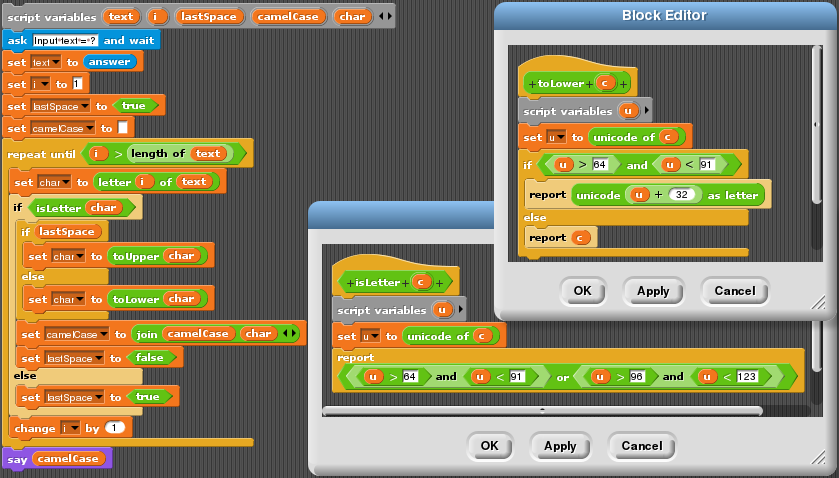CamelCase in Snap!

Top 10 users: